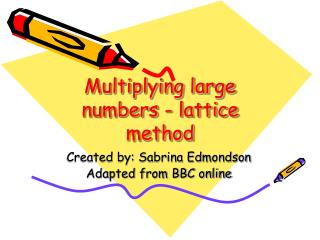DownloadDownload PresentationMultiplying large numbers - lattice method

# Multiplying large numbers - lattice method

Download Presentation## Multiplying large numbers - lattice method

- - - - - - - - - - - - - - - - - - - - - - - - - - - E N D - - - - - - - - - - - - - - - - - - - - - - - - - - -
##### Presentation Transcript

1. Multiplying large numbers - lattice method Created by: Sabrina Edmondson Adapted from BBC online

2. Multiplying large numbers - lattice method This method is also called Napier's bones. In some ways it is similar to the grid method as it involves multiplying rows and columns, but it gives you a better way to add the results at the end of the calculation.

3. Multiplying large numbers - lattice method With this method, you multiply each number in the column by each number in the row. Then you add along the diagonals to get the answer. Have a look at this equation: 25 x 5

4. Multiplying large numbers - lattice method Step 1: Make a grid with diagonal lines as shown below. Split the 25 into a 2 and a 5, putting each digit at the top of a column. Put the 5 by the top row. 25

5. Multiplying large numbers - lattice method Step 2: Multiply the columns by the rows, splitting the digits up on either side of the diagonal as shown here.

6. Multiplying large numbers - lattice method Step 3: Finally, add along the diagonals to get your answer. So: 25 x 5 = 125

7. Multiplying large numbers - lattice method This method is a bit complicated. Talking yourself through each step as you do it can be useful - someone is sure to ask you what you're doing and you'll end up explaining to them!

8. Multiplying large numbers - lattice method Let’s look at another multiplication equation worked out using the lattice method. With this method, you multiply each number in the column by each number in the row. Then you add along the diagonals to get the answer. Have a look at this equation: 25 x 5

9. Multiplying large numbers - lattice method 255 Step 1: Make a grid with diagonal lines as shown. Split the 255 into 2, 5, and 5, putting each digit at the top of a column. Split the 25 into 2 and 5, putting each digit by a row. 25

10. and 4 ones Multiplying large numbers - lattice method Step 2: Multiply the columns by the rows, splitting the digits up on either side of the diagonal as shown here.

11. Multiplying large numbers - lattice method Step 3: Finally, add along the diagonals to get your answer. So: 255 x 25 = 6375